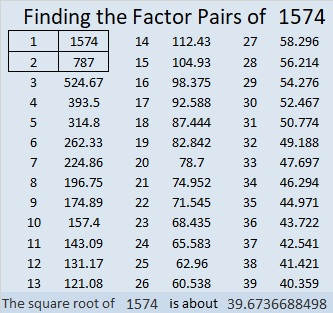# 1574 Avoiding Fractions When Using the Substitution Method to Solve Simultaneous Equations

Contents

### The Substitution Method

Jo Morgan examines six methods of solving simultaneous linear equations in her wonderful book A Compendium of Mathematical Methods. Six is two more than the number of methods I had known previously. Jo loves pouring over vintage mathematics books to learn how concepts were taught in days gone by. She loves finding resources on Twitter to teach concepts and describes herself as a Resourceaholic. Today is her birthday, and this post is dedicated to her.

In my experience, the substitution method is often the first method taught to solve simultaneous linear equations, and usually, before the accompanying homework assignment is finished, fractions will be part of the solution process. Once students learn other methods, they usually abandon the substitution method with its troublesome fractions. However, the substitution method is really just as good as any other method, and fractions can actually be avoided when using it! I encourage students to use the substitution method and avoid fractions while they work.

In the table below I use the substitution method twelve ways to find one coordinate of the solution. (In each case the other coordinate could be found by substituting the known value back into one of the original equations and solving for the other coordinate.) Notice that only the first column uses fractions; the other two columns do not.Follow each solution process step by step. Do any of the methods seem less confusing than the others? How will you approach the problem the next time you are asked to solve simultaneous linear equations?

### Factors of 1574:

This is my 1574th post. Here is some information about that number:

• 1574 is a composite number.
• Prime factorization: 1574 = 2 × 787.
• 1574 has no exponents greater than 1 in its prime factorization, so √1574 cannot be simplified.
• The exponents in the prime factorization are 1 and 1. Adding one to each exponent and multiplying we get (1 + 1)(1 + 1) = 2 × 2 = 4. Therefore 1574 has exactly 4 factors.
• The factors of 1574 are outlined with their factor pair partners in the graphic below.### Another Fact about the Number 1574:

1574 is in just one Pythagorean triple:
1574-619368-619370, calculated from 2(787)(1), 787²-1², 787²+1².
It can also be calculated from 2(394²-393²), 4(394)(393), 2(394²+393²).

This site uses Akismet to reduce spam. Learn how your comment data is processed.